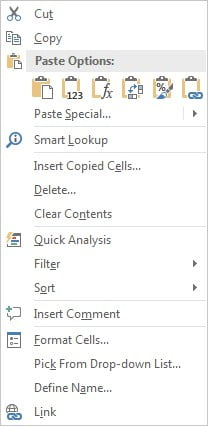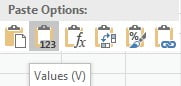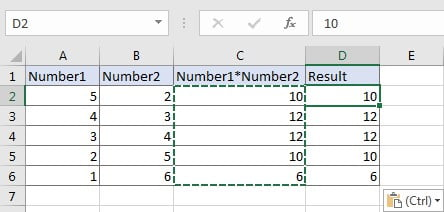# How to Copy and Paste Only Values and Ignore Formula

When we copy a cell applied with a formula, we copy the formula of the cell rather than copy the value showing in the cell. In this article we will introduce you the way to copy only value ignoring applied formula.

## EXAMPLE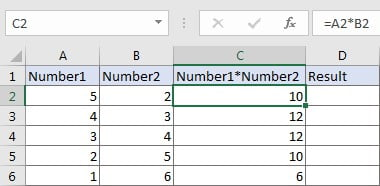In above table, column C records the product of number1 multiples number2. The product is calculated by formula =A*B, this formula is applied for cells in range C2:C6. If we want to copy these products from C2:C6 to D2:D6, can we just copy and paste them directly?

In fact, if we just select rangeC2:C6, copy and paste them into D2:D6, products of B*C are displayed automatically, that’s because when copying the values, the applied formulas in these cells are copied instead of the final results. So, when pasting these values into D2:D6, we actually paste the formula into these cells, and cell references in the formula are auto updated due to formula is copied to its adjacent cell in the same row.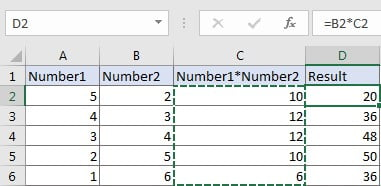## RESOLUTION

Excel Paste function provides many paste options like paste values, paste formatting, paste formulas when pasting data. In this instance, you can choose paste option “Values” when pasting data, then only values are kept.

## STEPS

a. Select range C2:C6, press Ctrl+C.